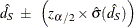#### Common Risk Difference

PROC FREQ provides Mantel-Haenszel and summary score estimates for the common risk (proportion) difference for multiwaytables.

##### Mantel-Haenszel Estimate of the Common Risk Difference

PROC FREQ computes the Mantel-Haenszel (Mantel and Haenszel, 1959) estimate of the common risk difference as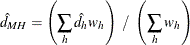where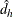is the risk difference in stratum h and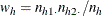The column 1 risk difference in stratum h is computed as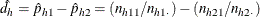where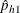is the proportion of row 1 observations that are classified in column 1 and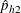is the proportion or row 2 observations that are classified in column 1. The column 2 risk is computed in the same way. For more information, see Agresti (2013, p. 231).

PROC FREQ computes the variance of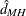(Sato, 1989) as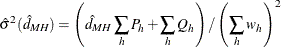where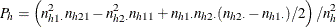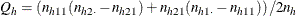The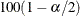% confidence limits for the common risk difference are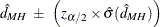##### Summary Score Estimate of the Common Risk Difference

PROC FREQ computes the summary score estimate of the common risk difference as described in Agresti (2013, p. 231). This estimate is computed from Miettinen-Nurminen (score) confidence limits for the stratum risk differences. For more information, see the section Miettinen-Nurminen (Score) Confidence Limits. The score confidence interval for the risk difference in stratum h can be expressed as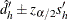, where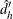is the midpoint of the score confidence interval and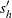is the width of the confidence interval divided by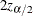. The summary score estimate of the common risk difference is computed as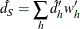where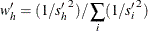The variance of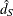is computed as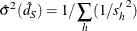The% summary score confidence limits for the common risk difference are